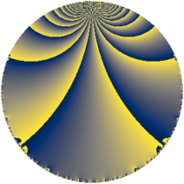# Properties

 Label 48.4.kLevel $48$ Weight $4$ Character orbit 48.k Rep. character $\chi_{48}(11,\cdot)$ Character field $\Q(\zeta_{4})$ Dimension $44$ Newform subspaces $1$ Sturm bound $32$ Trace bound $0$

# Related objects

## Defining parameters

 Level: $$N$$ $$=$$ $$48 = 2^{4} \cdot 3$$ Weight: $$k$$ $$=$$ $$4$$ Character orbit: $$[\chi]$$ $$=$$ 48.k (of order $$4$$ and degree $$2$$) Character conductor: $$\operatorname{cond}(\chi)$$ $$=$$ $$48$$ Character field: $$\Q(i)$$ Newform subspaces: $$1$$ Sturm bound: $$32$$ Trace bound: $$0$$

## Dimensions

The following table gives the dimensions of various subspaces of $$M_{4}(48, [\chi])$$.

Total New Old
Modular forms 52 52 0
Cusp forms 44 44 0
Eisenstein series 8 8 0

## Trace form

 $$44 q - 2 q^{3} - 4 q^{4} + 28 q^{6} - 8 q^{7} + O(q^{10})$$ $$44 q - 2 q^{3} - 4 q^{4} + 28 q^{6} - 8 q^{7} + 56 q^{10} - 80 q^{12} - 4 q^{13} - 112 q^{16} + 52 q^{18} + 20 q^{19} - 56 q^{21} - 40 q^{22} - 120 q^{24} - 134 q^{27} - 296 q^{28} - 332 q^{30} - 4 q^{33} + 520 q^{34} - 604 q^{36} - 4 q^{37} + 596 q^{39} + 632 q^{40} + 696 q^{42} - 436 q^{43} - 252 q^{45} + 664 q^{46} + 1200 q^{48} + 972 q^{49} - 648 q^{51} + 320 q^{52} + 1592 q^{54} + 280 q^{55} - 424 q^{58} + 800 q^{60} - 916 q^{61} - 2056 q^{64} - 668 q^{66} - 1636 q^{67} + 52 q^{69} - 5192 q^{70} - 3704 q^{72} + 1454 q^{75} - 568 q^{76} - 4932 q^{78} - 4 q^{81} + 768 q^{82} - 2096 q^{84} + 736 q^{85} + 1284 q^{87} + 8864 q^{88} + 2672 q^{90} + 424 q^{91} - 2084 q^{93} + 5616 q^{94} + 8008 q^{96} - 8 q^{97} + 1196 q^{99} + O(q^{100})$$

## Decomposition of $$S_{4}^{\mathrm{new}}(48, [\chi])$$ into newform subspaces

Label Dim $A$ Field CM Traces $q$-expansion
$a_{2}$ $a_{3}$ $a_{5}$ $a_{7}$
48.4.k.a $44$ $2.832$ None $$0$$ $$-2$$ $$0$$ $$-8$$